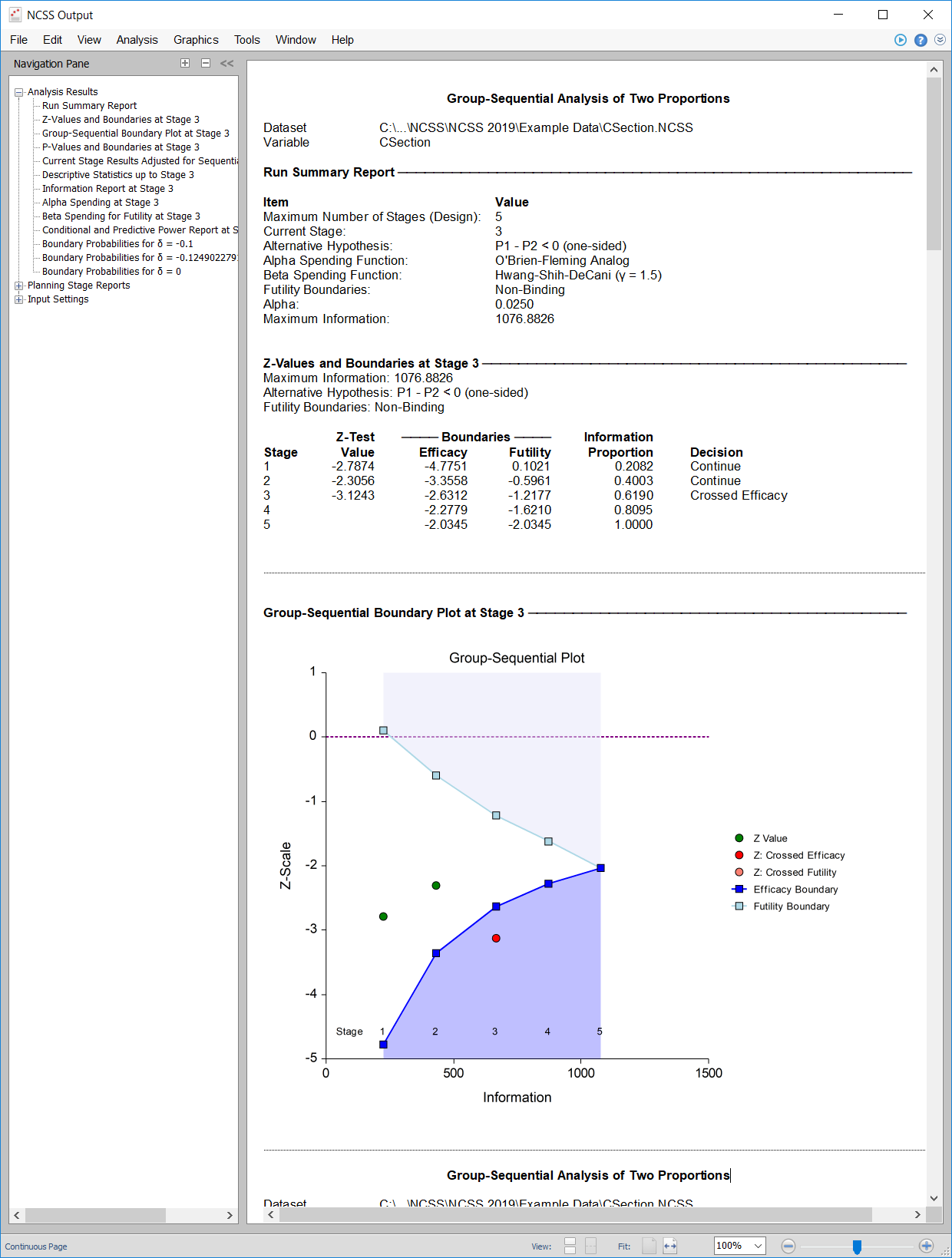# Group-Sequential Analysis in NCSS

NCSS includes tools for performing group-sequential analysis of one or two means, two proportions, and two hazard rates. Tools are also available for non-inferiority and superiority by a margin tests. The group-sequential analysis procedures in NCSS are easy-to-use and validated for accuracy. Use the links below to jump to the group-sequential analysis topic you would like to examine. To see how these tools can benefit you, we recommend you download and install the free trial of NCSS. For details about the initial sample size estimation in PASS, as well as other general group-sequential testing details, click here.

## Introduction

When data is gathered over time, it can be useful to make statistical comparisons when only portions of the data have been collected. Group-sequential analysis permits one to evaluate significant statistical difference at any stage in data collection. To accommodate this, and to maintain proper error control, the specified alpha and/or beta is apportioned according to appropriate alpha- or beta-spending functions. Boundaries for each stage are determined by the amount of data collected to that point (the information) and the amount of alpha or beta spent, as determined by the spending function(s). A group-sequential plot may be used to show the current progress of a group-sequential analysis.Boundaries may be determined for either efficacy (significant difference), or futility (lack of evidence of difference), or both (as shown in the plot).

## Technical Details

For in depth details of a group-sequential analysis, we refer the reader to the 'Documentation PDF' of each procedure, along with the referenced materials in that documentation. A brief outline is presented here.

### Design Phase

In the design phase, an initial calculation of the sample size needed (if the final stage is reached) is made. Corresponding to each NCSS procedure for group-sequential analysis, there exists a companion procedure in PASS Sample Size software for making this calculation. This calculation requires the input of a number of parameters, including desired alpha, power, boundary types, maximum number of stages, spending functions, and assumed differences, among others. We refer the user to the PASS software and corresponding documentation for more details on sample size estimation.

### Group-Sequential Analysis Phase

The design phase gives the target number of subjects for the first stage. The study begins, and response data is collected for subjects, moving toward the first-stage target number of subjects, until a decision to perform an analysis on the existing data is made.The analysis at this point is called the first stage.A z-statistic (or in some cases t-) is calculated from the raw data. The stage one z-statistic is compared to each of the stage one boundaries. Typically, if one of the boundaries is crossed, the study is stopped (non-binding futility boundaries may be an exception). If none of the boundaries are crossed the study continues to the next stage. If none of the boundaries are crossed it may also be useful to examine the conditional power or stopping probabilities of future stages. Conditional power and stopping probabilities are based on the user-specified supposed true difference. If the boundaries are not crossed, a revised target sample size is estimated, and the study continues until the decision is made to perform the next interim analysis on the cumulative response data. In the same manner as the first stage, the current stage information proportion is used with the spending function to determine alpha and/or beta spent at the current stage. The current stage boundaries are then computed. The z-statistic is calculated and compared to the boundaries, and a decision is made to stop or continue. The study continues from stage to stage until the study is stopped for the crossing of a boundary, or until the final stage is reached. If the final stage is reached a decision must be made, since the final stage efficacy and futility boundaries are the same value.### Reporting Phase

Once a group-sequential boundary is crossed and the decision is made to stop, there remains the need to properly summarize and communicate the study results. Some or all of the following may be reported: Boundary plot showing the crossed boundary; Adjusted confidence interval and estimate of the mean difference; Sample size used

## Group-Sequential Analysis for Two Means with Known Variances

[Documentation PDF]

Because the group variances are rarely known in practice, this procedure may be more academic in nature. An understanding of this procedure can be helpful in better understanding the similar procedure based on T-Tests. The corresponding PASS Sample Size procedure is Group-Sequential Tests for Two Means with Known Variances (Simulation).

## Group-Sequential T-Tests for Two Means

[Documentation PDF]

In this procedure, the underlying z-score calculations are used to produce corresponding t-statistic boundary calculations. Simulation-based sample-size re-estimation at each stage is a very useful part of this procedure. The corresponding PASS Sample Size procedure is Group-Sequential T-Tests for Two Means (Simulation).

## Group-Sequential Analysis for Two Proportions

[Documentation PDF]

This procedure gives group-sequential tests for the common Wald Z-test, using the unpooled variance estimate, with or without the continuity correction. Simulation-based sample-size re-estimation at each stage is a very useful part of this procedure. The corresponding PASS Sample Size procedure is Group-Sequential Tests for Two Proportions (Simulation).

## Group-Sequential Analysis for Two Hazard Rates

[Documentation PDF]

This procedure gives group-sequential tests for the difference of two hazard rates, assuming an underlying Exponential model. Simulation-based sample-size re-estimation at each stage is a very useful part of this procedure. The corresponding PASS Sample Size procedure is Group-Sequential Tests for Two Hazard Rates (Simulation).

"Your [PASS] routines are flexible and easy to use and your customer service and support is A-double-plus."

Nayak Polissar, PhD

"PASS 13 is fantastic! Better than my new dishwasher and microwave combined."

Dr. Barbara Tabachnick, CSUN, Author